CUET  >  Water Images Quiz II, Non Verbal Reasoning

# Water Images Quiz II, Non Verbal Reasoning - CUET

Test Description

## 20 Questions MCQ Test General Test Preparation for CUET - Water Images Quiz II, Non Verbal Reasoning

Water Images Quiz II, Non Verbal Reasoning for CUET 2023 is part of General Test Preparation for CUET preparation. The Water Images Quiz II, Non Verbal Reasoning questions and answers have been prepared according to the CUET exam syllabus.The Water Images Quiz II, Non Verbal Reasoning MCQs are made for CUET 2023 Exam. Find important definitions, questions, notes, meanings, examples, exercises, MCQs and online tests for Water Images Quiz II, Non Verbal Reasoning below.
Solutions of Water Images Quiz II, Non Verbal Reasoning questions in English are available as part of our General Test Preparation for CUET for CUET & Water Images Quiz II, Non Verbal Reasoning solutions in Hindi for General Test Preparation for CUET course. Download more important topics, notes, lectures and mock test series for CUET Exam by signing up for free. Attempt Water Images Quiz II, Non Verbal Reasoning | 20 questions in 40 minutes | Mock test for CUET preparation | Free important questions MCQ to study General Test Preparation for CUET for CUET Exam | Download free PDF with solutions
 1 Crore+ students have signed up on EduRev. Have you?
Water Images Quiz II, Non Verbal Reasoning - Question 1

### Directions to Solve In each of the following questions, choose the water image of the Fig.(X) from amongst the four alternatives (1), (2), (3) and (4) given along with it. Question - Choose the correct water image of the given figure (X) from amongst the four alternatives.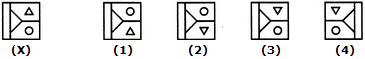Water Images Quiz II, Non Verbal Reasoning - Question 2

### Directions to Solve In each of the following questions, choose the water image of the Fig.(X) from amongst the four alternatives (1), (2), (3) and (4) given along with it. Question - Choose the correct water image of the given figure (X) from amongst the four alternatives.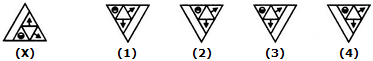Water Images Quiz II, Non Verbal Reasoning - Question 3

### Directions to Solve In each of the following questions, choose the water image of the Fig.(X) from amongst the four alternatives (1), (2), (3) and (4) given along with it. Question - Choose the correct water image of the given figure (X) from amongst the four alternatives.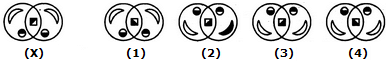Water Images Quiz II, Non Verbal Reasoning - Question 4

In each of the following questions, choose the water image of the Fig.(X) from amongst the four alternatives (1), (2), (3) and (4) given along with it.

Question -

Choose the correct water image of the given figure (X) from amongst the four alternatives.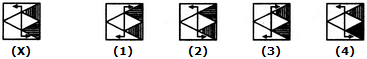Water Images Quiz II, Non Verbal Reasoning - Question 5

In each of the following questions, choose the water image of the Fig.(X) from amongst the four alternatives (1), (2), (3) and (4) given along with it.

Question -

Choose the correct water image of the given figure (X) from amongst the four alternatives.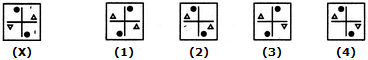Water Images Quiz II, Non Verbal Reasoning - Question 6

In each of the following questions, choose the water image of the Fig.(X) from amongst the four alternatives (1), (2), (3) and (4) given along with it.

Question -

Choose the correct water image of the given figure (X) from amongst the four alternatives.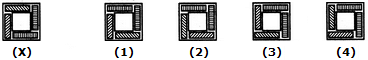Water Images Quiz II, Non Verbal Reasoning - Question 7

In each of the following questions, choose the water image of the Fig.(X) from amongst the four alternatives (1), (2), (3) and (4) given along with it.

Question -

Choose the correct water image of the given figure (X) from amongst the four alternatives.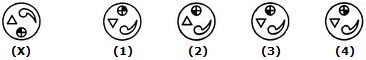Water Images Quiz II, Non Verbal Reasoning - Question 8

In each of the following questions, choose the water image of the Fig.(X) from amongst the four alternatives (1), (2), (3) and (4) given along with it.

Question -

Choose the correct water image of the given figure (X) from amongst the four alternatives.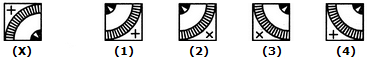Water Images Quiz II, Non Verbal Reasoning - Question 9

In each of the following questions, choose the water image of the Fig.(X) from amongst the four alternatives (1), (2), (3) and (4) given along with it.

Question -

Choose the correct water image of the given figure (X) from amongst the four alternatives.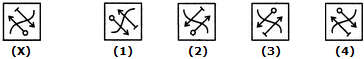Water Images Quiz II, Non Verbal Reasoning - Question 10

In each of the following questions, choose the water image of the Fig.(X) from amongst the four alternatives (1), (2), (3) and (4) given along with it.

Question -

Choose the correct water image of the given figure (X) from amongst the four alternatives.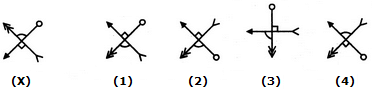Water Images Quiz II, Non Verbal Reasoning - Question 11

In each of the following questions, choose the water image of the Fig.(X) from amongst the four alternatives (1), (2), (3) and (4) given along with it.

Question -

Choose the correct water image of the given figure (X) from amongst the four alternatives.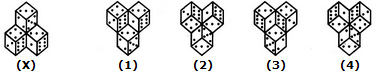Water Images Quiz II, Non Verbal Reasoning - Question 12

In each of the following questions, choose the water image of the Fig.(X) from amongst the four alternatives (1), (2), (3) and (4) given along with it.

Question -

Choose the correct water image of the given figure (X) from amongst the four alternatives.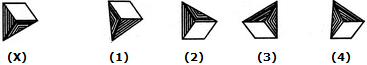Water Images Quiz II, Non Verbal Reasoning - Question 13

In each of the following questions, choose the water image of the Fig.(X) from amongst the four alternatives (1), (2), (3) and (4) given along with it.

Question -

Choose the correct water image of the given figure (X) from amongst the four alternatives.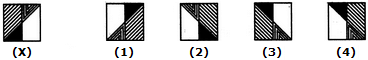Water Images Quiz II, Non Verbal Reasoning - Question 14

In each of the following questions, choose the water image of the Fig.(X) from amongst the four alternatives (1), (2), (3) and (4) given along with it.

Question -

Choose the correct water image of the given figure (X) from amongst the four alternatives.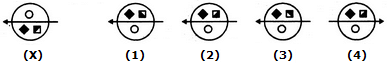Water Images Quiz II, Non Verbal Reasoning - Question 15

In each of the following questions, choose the water image of the Fig.(X) from amongst the four alternatives (1), (2), (3) and (4) given along with it.

Question -

Choose the correct water image of the given figure (X) from amongst the four alternatives.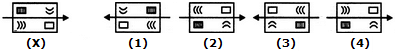Water Images Quiz II, Non Verbal Reasoning - Question 16

In each of the following questions, choose the water image of the Fig.(X) from amongst the four alternatives (1), (2), (3) and (4) given along with it.

Question -

Choose the correct water image of the given figure (X) from amongst the four alternatives.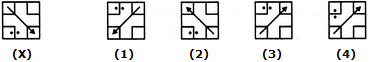Water Images Quiz II, Non Verbal Reasoning - Question 17

In each of the following questions, choose the water image of the Fig.(X) from amongst the four alternatives (1), (2), (3) and (4) given along with it.

Question -

Choose the correct water image of the given figure (X) from amongst the four alternatives.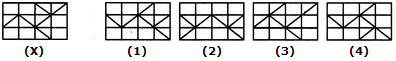Water Images Quiz II, Non Verbal Reasoning - Question 18

In each of the following questions, choose the water image of the Fig.(X) from amongst the four alternatives (1), (2), (3) and (4) given along with it.

Question -

Choose the correct water image of the given figure (X) from amongst the four alternatives.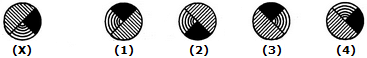Water Images Quiz II, Non Verbal Reasoning - Question 19

In each of the following questions, choose the water image of the Fig.(X) from amongst the four alternatives (1), (2), (3) and (4) given along with it.

Question -

Choose the correct water image of the given figure (X) from amongst the four alternatives.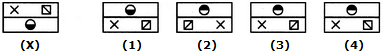Water Images Quiz II, Non Verbal Reasoning - Question 20

In each of the following questions, choose the water image of the Fig.(X) from amongst the four alternatives (1), (2), (3) and (4) given along with it.

Question -

Choose the correct water image of the given figure (X) from amongst the four alternatives.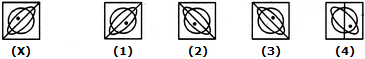## General Test Preparation for CUET

176 videos|328 docs|399 tests
Information about Water Images Quiz II, Non Verbal Reasoning Page
In this test you can find the Exam questions for Water Images Quiz II, Non Verbal Reasoning solved & explained in the simplest way possible. Besides giving Questions and answers for Water Images Quiz II, Non Verbal Reasoning, EduRev gives you an ample number of Online tests for practice

## General Test Preparation for CUET

176 videos|328 docs|399 tests